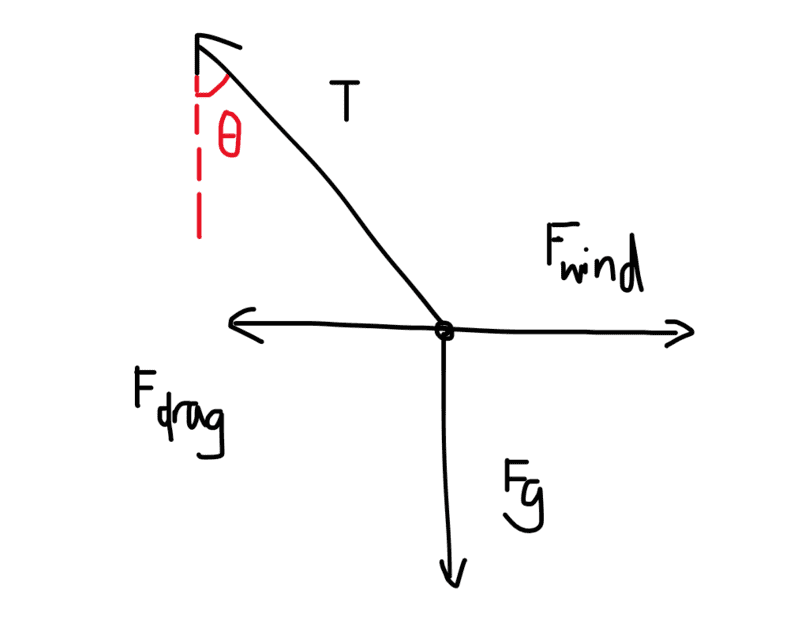# Wind drag problem with a ball hanging on a rope

crememars
Homework Statement:
A 1.2 kg ball is hanging from the end of a rope. The rope hangs at an angle of 25 degrees from the vertical when a 15.0m/s horizontal wind is blowing. If the wind’s force on the rope is negligible, what drag force does the wind exert on the ball?
Relevant Equations:I drew a FBD but I feel like it's wrong because there are too many missing values. I tried this:

Fy = 0
Tcosθ - Fg = 0
Tcosθ = mg
T = (1.2)(9.8) / cos(25)
T = 12.98 N

Fx = ma
Fwind - Tsinθ - Fdrag = ma
Fwind - (12.98)(sin25) - Fdrag = ma

I don't know how to find these missing values. I feel like I'm approaching the situation wrong? I saw one solution solve it like this:

g(tanθ) = a
(9.8)(tan25) = 4.57

Fx = ma
Fx = (1.2)(4.57)
Fx = 5.48 N

but this doesn't make any sense to me.

any help would be appreciated, thank you !

Gold Member
MHB
Homework Statement:: A 1.2 kg ball is hanging from the end of a rope. The rope hangs at an angle of 25 degrees from the vertical when a 15.0m/s horizontal wind is blowing. If the wind’s force on the rope is negligible, what drag force does the wind exert on the ball?
Relevant Equations:: the answer is 5.84 N

View attachment 315675
I drew a FBD but I feel like it's wrong because there are too many missing values. I tried this:

Fy = 0
Tcosθ - Fg = 0
Tcosθ = mg
T = (1.2)(9.8) / cos(25)
T = 12.98 N

Fx = ma
Fwind - Tsinθ - Fdrag = ma
Fwind - (12.98)(sin25) - Fdrag = ma

I don't know how to find these missing values. I feel like I'm approaching the situation wrong? I saw one solution solve it like this:

g(tanθ) = a
(9.8)(tan25) = 4.57

Fx = ma
Fx = (1.2)(4.57)
Fx = 5.48 N

but this doesn't make any sense to me.

any help would be appreciated, thank you !
You have too many forces on your diagram. The force from the wind is the force of the drag. So you only have the force from the wind acting to the right.

-Dan

•SammyS, crememars and erobz
crememars
You have too many forces on your diagram. The force from the wind is the force of the drag. So you only have the force from the wind acting to the right.

-Dan
I think I understood, thank you! so the drag would be equal to the x component of the tension.